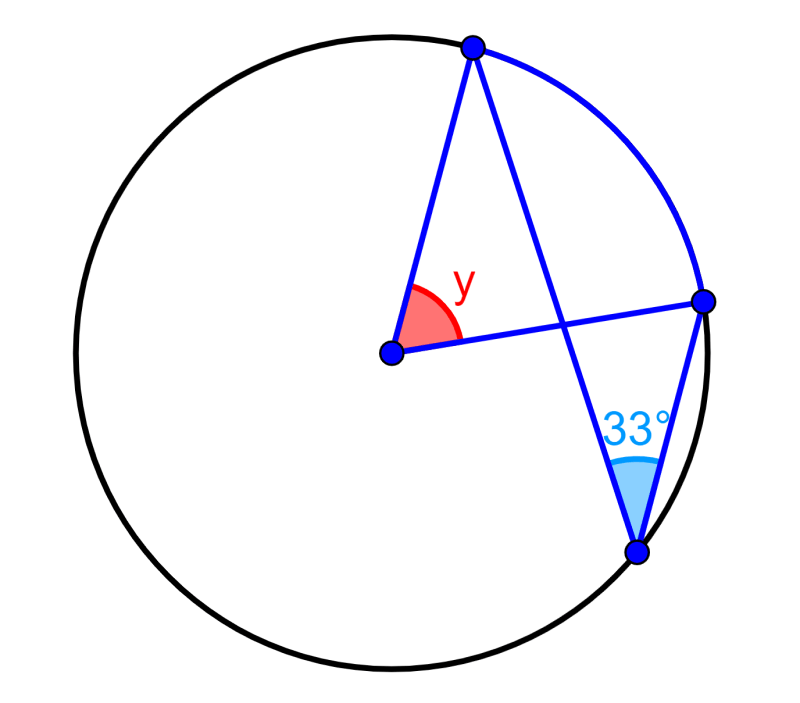# Inscribed Angle Theorem – Formulas and Examples

The inscribed angle theorem is one of the most important theorems in geometry. This theorem tells us that when we have a central angle and an inscribed angle with the same endpoints, the size of the central angle is twice the size of the inscribed angle. This theorem has three different variations depending on the location of the angles.

##### GEOMETRY

Relevant for

Learning about the inscribed angle theorem with examples.

See theorem

##### GEOMETRY

Relevant for

Learning about the inscribed angle theorem with examples.

See theorem

## What are inscribed angles?

Inscribed angles are angles that have vertices that lie inside a circle. The two sides of an inscribed angle are chords of the circle.

Additionally, we also have the central angles, which are located in the center of the circle and their sides are formed by two radii of the circle.

The angle formed by the ends of two chords on the circumference of a circle is called the intercepted arc.

We can look at these three angles in the following diagram:

Therefore, we have:

α = central angle

β = inscribed angle

θ = intercepted arc

## What is the inscribed angle theorem?

The inscribed angle theorem, also known as the central angle theorem, tells us the following:

Every time the central angle and the inscribed angle share endpoints on the circle, the value of the central angle is twice that of the inscribed angle.

Therefore, the inscribed angle theorem is represented by the following formula:

α = 2β

where α is the central angle and β is the inscribed angle.

## Cases and proof of the inscribed angle theorem

We have three different cases depending on the location of the angles:

• When the diameter is located between the sides of the inscribed angle.
• When the inscribed angle is located between a chord and the diameter of the circle.
• When the diameter is located outside the sides of the inscribed angle.

We can look at the three cases in the following diagram:

The three cases are equivalent since all the inscribed angles that delimit in the same arc are equal as shown in the following diagram:

Detailed proofs of each of these cases can be found in this article.

## Inscribed angle theorem – Examples with answers

### EXAMPLE 1

Determine the size of angle x in the following diagram:

Solution: By the inscribed angle theorem, we know that the central angle is twice the inscribed angle. Therefore, we have: :

central angle = 2×inscribed angle

central angle = 2×50°

central angle = 100°

### EXAMPLE 2

The green angle has its vertex in the center of the circle. What is the value of x?

Solution: The green angle with a size of x+50° is the central angle for the inscribed angle x since they both intersect the same arc. Therefore, the size of the green angle is twice the size of the red angle. Then, we have:

2x=x+50°

x=50°

### EXAMPLE 3

Find the value of x in the following diagram:

Solution: We have that the central angle has a value of 48°. By the inscribed angle theorem, we have:

2x=48°

We divide both sides by 2 to get:

x=24°

### EXAMPLE 4

What is the value of x+y?

Solution: We know that the triangle containing the red angle and the green angle is an isosceles triangle since two of the sides are the radii of the circle. Also, we know that the interior angles of any triangle add up to 180°. Therefore, we can find the size of the red angle as follows:

110°+x+x=180°

2x=180°-110°

2x=70°

x=35°

Now, we know that the angle y is the central angle, so it must be twice the inscribed angle and we have:

y=2x

y=2(35°)

y=70°

## Inscribed angle theorem – Practice problems

#### What is the size of angle x?#### Determine the size of the angle y.#### What is the result of x+y?Interested in learning more about the inscribed angle theorem and other angles? Take a look at these pages:### Jefferson Huera Guzman

Jefferson is the lead author and administrator of Neurochispas.com. The interactive Mathematics and Physics content that I have created has helped many students.